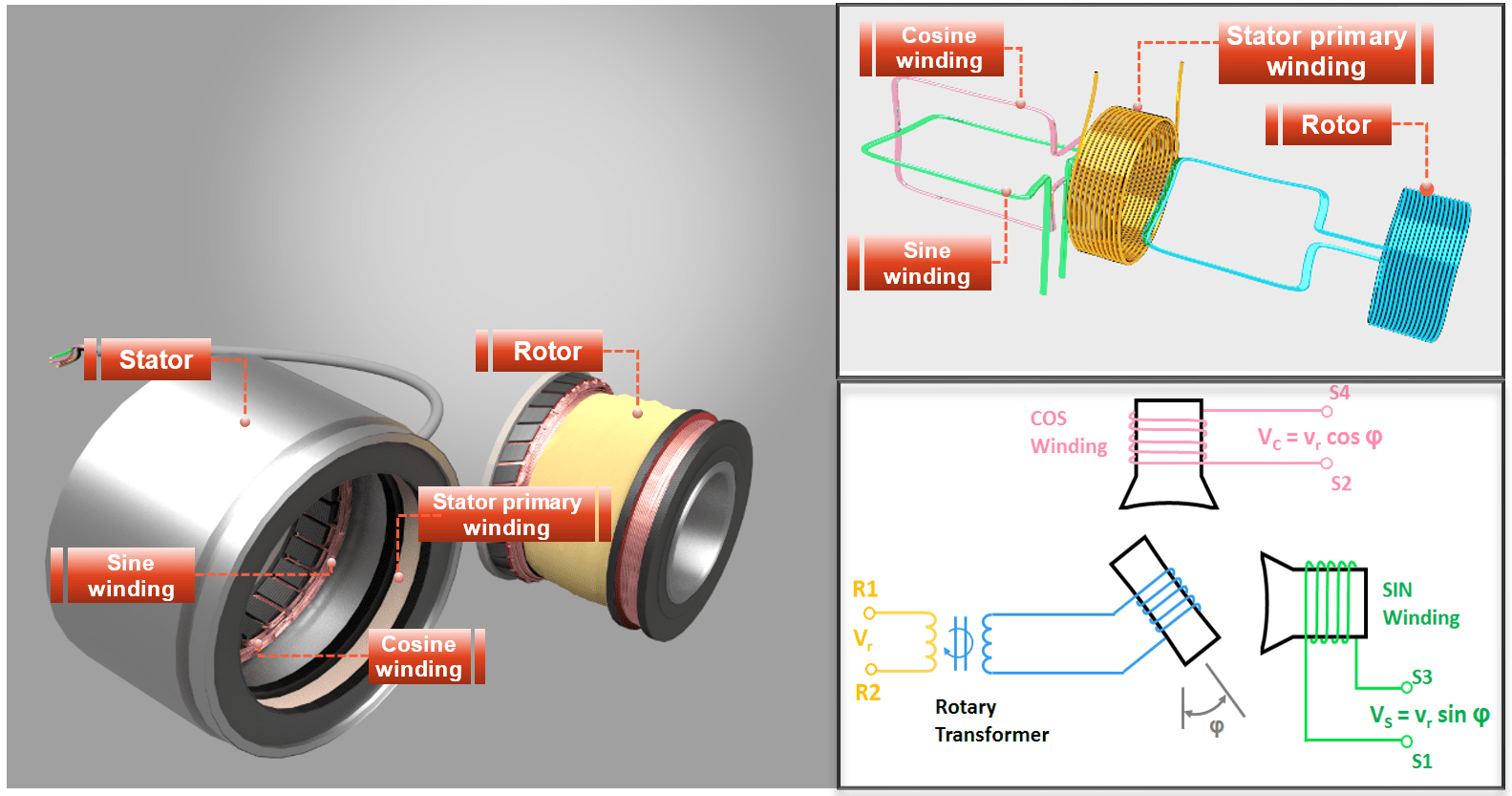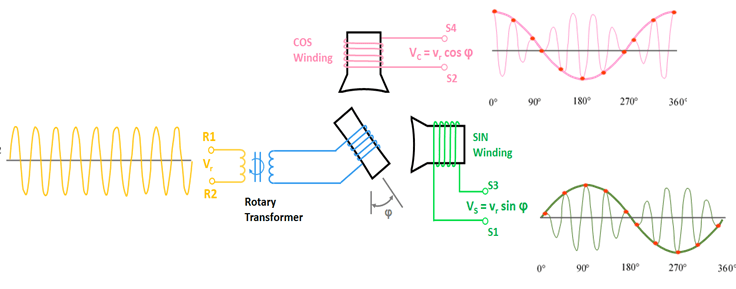# Resolver### How does a resolver work

We are proud to present you this video about the resolver functioning:

The resolver consists of a stationary part called stator, and a revolving part called rotor, which is attached to the motor shaft.Resolver - Function

Function: The primary winding of the stator is connected to a high frequency sine signal. This sine signal is transmitted to rotor winding, because the stator´s primary winding and the rotor winding act together like a transformer. Furthermore, we can call the rotor winding the Reference winding.

The pulsating alternating magnetic field of the rotor winding now induces an alternating voltage in the measuring windings sine and cosine. Their amplitudes, however, depend on the angular position of the rotor.

If the rotor winding and the measuring winding are parallel to one another, the magnetic rotor field completely passes through the measuring coil and therefore the induced voltage is maximal.

However, if the rotor winding and measuring winding are at right angles to each other, no voltage is induced.

Resolver signals at high rpm:

The output signals are now a modulated sine and cosine signal. A graphical representation of the excitation signal and the sine and cosine output signals is shown below:Evaluating Resolver signal

Here shown the high-frequency excitation voltage (2kHz - 10kHz) applied to the stator primary winding. It is now up to the RDC (resolver digital converter) to evaluate from this signal curve the current rotor position and the rotational speed. The calculation formulas are as follows:

Input signal for the stator primary winding:
UR1-R2 = A sin (ω t)

Peak value of the amplitudes:
US1 = k * A * sin α * sin (ω t + φ)
US2 = k * A * cos α * sin (ω t + φ)

Angular position:
α = arctan US1 / US2

UR1-R2 = Reference signal at the stator winding
US1 = Measuring signal at the sine winding
US2 = Measuring signal at the cosine winding
k = Transformation ratio between Input signal and measuring signal
φ = Phase shift between input and output voltage

Resolver vs Encoder: Unlike Encoders, Resolvers have no electronic components and therefore robust against soiling, vibrations and works safely even within a wide temperature range. This makes them highly reliable and environmentally resistant.